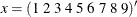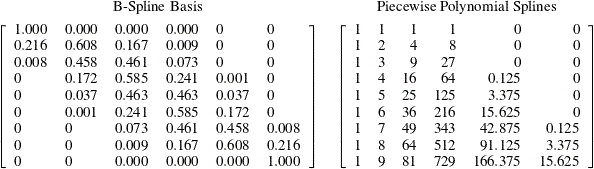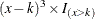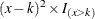# The TRANSREG Procedure

### SPLINE and MSPLINE Transformations

The missing portions of variables subjected to SPLINE or MSPLINE transformations are handled the same way as for OPSCORE , MONOTONE , UNTIE , and LINEAR transformations (see the previous section). The nonmissing partition is handled by first creating a B-spline basis of the specified degree with the specified knots for the nonmissing partition of the initial scaling vector and then regressing the target onto the basis. The optimally scaled vector is a linear combination of the B-spline basis vectors. Ordinary least squares regression coefficients are used. An algorithm for generating the B-spline basis is given in De Boor (1978, pp. 134–135). B-splines are both a computationally accurate and efficient way of constructing a basis for piecewise polynomials; however, they are not the most natural method of describing splines.

Consider an initial scaling vectorand a degree-three spline with interior knots at 3.5 and 6.5. The B-spline basis for the transformation is the left matrix, and the natural piecewise polynomial spline basis is the right matrix.The two matrices span the same column space. The natural basis has an intercept, a linear term, a quadratic term, a cubic term, and two more terms since there are two interior knots. These terms are generated (for knot k andelement x) by the formula. The indicator variableevaluates to 1.0 if x is greater than k and to 0.0 otherwise. If knot k had been repeated, there would be aterm also. Notice that the fifth column makes no contribution to the curve before 3.5, makes zero contribution at 3.5 (the transformation is continuous), and makes an increasing contribution beyond 3.5. The same pattern of results holds for the last term with knot 6.5. The coefficient of the fifth column represents the change in the cubic portion of the curve after 3.5. The coefficient of the sixth column represents the change in the cubic portion of the curve after 6.5.

The numbers in the B-spline basis do not have a simple interpretation like the numbers in the natural piecewise polynomial basis. The B-spline basis has a diagonally banded structure. The band shifts one column to the right after every knot. The number of entries in each row that can potentially be nonzero is one greater than the degree. The elements within a row always sum to one. The B-spline basis is accurate because of the smallness of the numbers and the lack of extreme collinearity inherent in the natural polynomials. B-splines are efficient because PROC TRANSREG can take advantage of the sparseness of the B-spline basis when it accumulates crossproducts. The number of required multiplications and additions to accumulate the crossproduct matrix does not increase with the number of knots but does increase with the degree of the spline, so it is much more computationally efficient to increase the number of knots than to increase the degree of the polynomial.

MSPLINE transformations are handled like SPLINE transformations except that constraints are placed on the coefficients to ensure monotonicity. When the coefficients of the B-spline basis are monotonically increasing, the transformation is monotonically increasing. When the polynomial degree is two or less, monotone coefficient splines, integrated splines (Winsberg and Ramsay, 1980), and the general class of all monotone splines are equivalent.